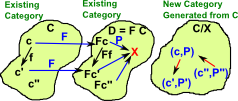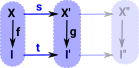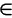# Category Theory - Arrow, Comma and Slice CategoriesOn a previous page we looked at ways to construct categories from existing categories. On this page we look at how objects in existing categories can become arrows in a new category.

A specific case of arrow categories is comma categories and a more specific case is slice categories. We can then further generalise to pullbacks.

In some circumstances we will see that certain universal properties are conserved.

## Arrow CategoryThe arrow category gives us a way to convert objects into arrows.
 objects: f: A -> X Objects in this arrow category are arrows between two categories. To specify this completely we need a triple consisting of the two objects and the morphism between them. morphisms: where 's' is an arrow in the source and 't' is an arrow in the target.

where the above diagram commutes, that is:

g•s = t •f

## Comma CategoryThe comma category is a specialisation of the arrow category where the codomain is the same for all objects. Alternatively there is also a cocomma category where the domain is the same for all objects.The above diagram could be rewritten with the X shown in each object to make it look more like the arrow category.

The category of graphs is an example of a comma category, that is, a graph is isomorphic to a comma category. Arrows of graphs are pairs of functions mapping nodes to nodes (N -> N) and edges to edges (E -> E) , an object of this category it a triple (E,f: E -> N×N,N) where f maps each edge to a source and target node.

#### Objects

pairs(A,f)

Where:

• 'A' is any object in C
• 'f' is a mapping from A to X:
 Af X
pairs(A,f)
 Xf A

#### Morphisms

The morphism f:
 s (A,f) -> (A',f')
Such that the diagram commutesThe morphism f:
 t (A,f) -> (A',f')
Such that the diagram commutes#### Initial Objects in Co-Comma

If C has a terminal object '1' exists then:

C/1 = C

If C has an initial object '0' exists then:

0/C = C

#### CoProducts in Co-Comma

In terms of the above 'pairs' we can construct a product:
 Take arbitrary elements: A,B,D and mappings of these to X s : A->X, t : B->X, μ : D->XWe can reduce the above diagram to:## Slice Category

This construction allows us to start with one category 'C' and generate a different category 'C/X' by fixing a given element 'X' in C. The elements in C/X are pairs (A,P) where AC and P is a morphism from A to X.

An example might be adding a distinguished point (the origin) to Euclidean space to give a vector space.In the following we have also explained 'co-slice' the dual concept in the yellow boxes on the right.

Given a category C we can 'slice' it over some object XC which we fix in C.

Comma category is a more general case of the slice category (slice category is a specific case of comma category). this time we fix a functor f from category C to category D with an object XD.

So we fix f: C->D and XD

#### Objects

pairs(cC,p:Fc->X)
 FcP X
Where
pairs(cC,p:X->Fc)
 XP Fc

#### Morphisms

 The morphism f: c->c'C Such that the diagram commutesThe morphism f: c->c'C Such that the diagram commutesWe can generalise this further by not fixing XInstead x is combined with the pair to give a triple.

#### Objects

triple(cC,xD,p)

Where:

• x is any element in D (no longer fixed)
 FcP X
triple(cC,xD,p)
 XP Fc

#### Morphisms

 The morphisms are: f: c->c'C g: x->x'D Such that the diagram commutesFurther generalisation, we add a third category 'E' like this:#### Objects

quad(cC,eE,FX,p)
 FcP X
Where x is any element in D (no longer fixed)

#### Morphisms

 The morphisms are: f: c->c'C g: x->x'D Such that the diagram commutesWhere I can, I have put links to Amazon for books that are relevant to the subject, click on the appropriate country flag to get more details of the book or to buy it from them.The Princeton Companion to Mathematics - This is a big book that attempts to give a wide overview of the whole of mathematics, inevitably there are many things missing, but it gives a good insight into the history, concepts, branches, theorems and wider perspective of mathematics. It is well written and, if you are interested in maths, this is the type of book where you can open a page at random and find something interesting to read. To some extent it can be used as a reference book, although it doesn't have tables of formula for trig functions and so on, but where it is most useful is when you want to read about various topics to find out which topics are interesting and relevant to you.